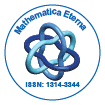# Mathematica EternaOpen Access

ISSN: 1314-3344

## Abstract

### q-Lie Algebras and q-3-Lie Algebras

BAI Ruipu, GUO Weiwei and LIN Lixin

In this paper, the quantum Lie algebras and quantum 3-Lie algebras over a field K with chK = 0 are discussed for q generic, where q ∈ K, q 6= 0, 1. A quantum Lie algebra is realized by a Z-graded algebra (Theorem 2.3), and a Lie algebra is realized by a quantum algebra which satisfying the property q −ixi(xjxk)q = (xixj )qxk (Theorem 2.4). From quantum Lie algebras and linear functions, two classes quantum 3-Lie algebras are constructed (Theorem 2.6 and Theorem 2.7).

Top